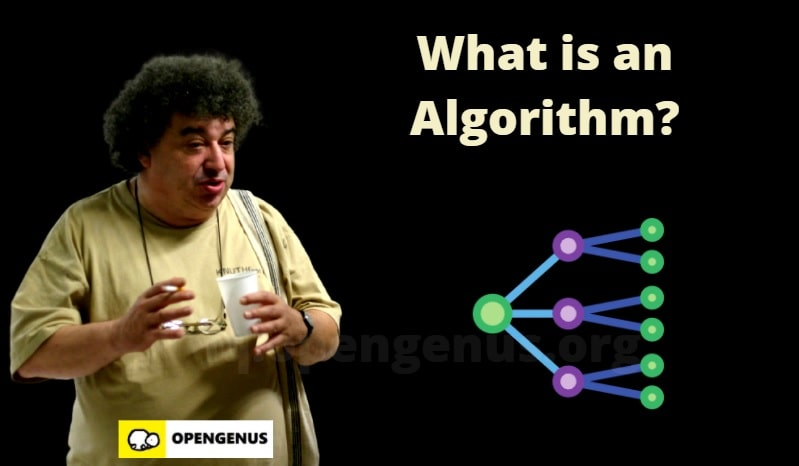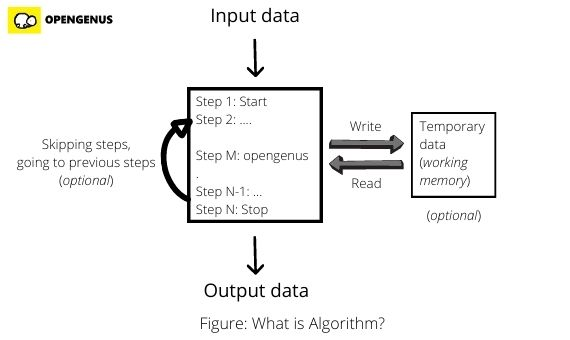×

Search anything:

# What is Algorithm ?

#### Algorithms CultureIn this article, we have explored what is algorithm (meaning of Algorithm) along with its technical definition, different types and other core Algorithmic ideas.

1. What is Algorithm?
2. Technical Definition of Algorithm in Computer Science
3. Different types of Algorithms
4. Are there Algorithms to solve every problem?
5. Applications of Algorithms
6. Conclusion## What is Algorithm?

Algorithm is an ordered well defined series of steps that can be followed to solve a problem. A problem can be finding a definitive answer or a yes or no decision.

An Algorithm may save temporary data which it might use during its steps. It may even take an input data to work on. Algorithm can be viewed as a set of instructions.

Examples of Algorithms:

• Sorting a list of numbers

There are several algorithms to sort a list of numbers. These algorithms take a list of numbers as input and the last step returns the list of sorted numbers. Examples of such algorithms are Quick Sort, Merge Sort and much more.

• Finding prime numbers

These algorithms may not need an input. It will continuously generate prime numbers. Additionally, these algorithms maintain a temporary data of potential numbers which it keeps updating to find large prime numbers at each step.

Example of such algorithms are Sieve of Eratosthenes and Sieve of Sundaram. The temporary data is known as sieve in these algorithms.Following is an example of an algorithm to find the difference of two numbers:

``````Step 1: Start
Step 2: Define two variables num1 and num2
Step 3: Get the inputs in the two variables num1 and num2
Step 4: Subtract two numbers and assign it to a new variable answer.
Step 5: Return the value of variable answer
Step 6: Stop
``````

Example of an algorithm to find factorial of a number N:

``````Step 1: Start
Step 2: Define a variable N
Step 3: Get the input in variable N
Step 4: Define a variable fact = 1
Step 5: If N >= 1, fact = fact * N
Step 6: Update N = N-1
Step 7: If N > 1, Go to step 5
Step 8: If N <= 1, Go to step 9
Step 9: Return the value of variable fact
Step 10: Stop
``````

Example of algorithm to find the larger of two numbers:

``````Step 1: Start
Step 2: Define two variables num1 and num2
Step 3: Get Input in the two variables num1 and num2
Step 4: If num1 > num2, return value of num1 and go to step 6
Step 5: return value of num2
Step 6: Stop
``````

An Algorithm can be implemented in a real system for use as:

• An implementation in a Programming Language
• A mechanical component in Analytical Computer
• A guideline to solve problems manually by Humans

## Technical Definition of Algorithm in Computer Science

The Technical Definition of Algorithm comes from the domain of "Theory of Computation" in a theorem named

A computation process that can be represented by an algorithm can be converted to a Turing Machine.

In simple terms, an algorithm is a series of steps that can be followed by a Turing Machine. A Turing Machine is a simple theoretical model consisting of a tape and pointer.

#### Algorithm = Church Turing Thesis

To solve the 10th Hilbert Problem, one needs to understand what is meant by an algorithm. In fact, there have been different definitions and all have proved to be equivalent. Some definitions were:

• 1936: Algorithm = Turing Machine
• 1936: Algorithm = Lambda Calculus
• 1970+: Algorithm = Implementation in Programming Languages like C and Lisp
• Final: Algorithm = process converted to Turing Machine.

Finally, it was agreed that an Algorithm is based on Church Turing Thesis which said:

"Any computational process can be considered as an Algorithm if it can be converted to a Turing Machine." Note: This does not hold true as of now.

Any Programming Language that is "Turing Complete" can be used to implement any Algorithm. This means if a Programming Language is not Turing Complete, then there are algorithms which cannot be implemented with it.

Example of Programming Languages that are not Turing Complete are SQL92, BNF, Regular expressions, Berkley Packet Filter (BPF) Bytecode, HTML, XML, JSON and YAML.

## Different types of Algorithms

There are Different types of Algorithms such as:

• Dynamic Programming Algorithm
• Backtracking Algorithm
• Greedy Algorithm
• Sorting Algorithm
• String Algorithm
• Mathematical Algorithm
• Recursive Algorithm
• Super Recursive Algorithm
• Divide and Conquer Algorithm
• Divide and Rule Algorithm
• Computational Geometry Algorithm

The algorithms are classified based on the core approach to solve problems.

Examples of prominent Algorithms are:

• Quick Sort
• Merge Sort
• Selection Sort
• Linear Search
• Binary Search
• Dijkstra's Algorithm
• Bellman Ford algorithm
• Floyd Warshall algorithm
• Johnson's algorithm
• A* search algorithm
• Boyer Moore String Search Algorithm
• Knuth Morris Pratt (KMP) Algorithm
• Boyer Moore Majority Vote algorithm

## Are there Algorithms to solve every problem?

Not all Computational problems can be solved by Algorithms. Based on two results "Church Turing Thesis" and "Godel's incompleteness theorem", there exist problems that stay out of reach of Algorithms.

In Computer Science, there exist a class of problems known as NP (Non-polynomial). These problems are difficult to be solved and there exist no optimal algorithm to solve them. In fact, it is proven that if one of the problems is solved by an algorithm efficiently, then all other problems can be solved with the same algorithm. There are algorithms to solve such problems but they would take centuries (>100 years) to run which makes them non-practical.

## Applications of Algorithms

Algorithms are the most important component of Computer Science. The core Applications of Algorithms are:

• In 1970s, Computing was declared as a domain of Science after several researchers demonstrated that analysis of Algorithms require significant knowledge and study. Previously, Computing was not considered to be a part of Science. Algorithms played a major role in bringing Computer Science to the mainstream.
• Algorithms are the basis of core functionality of several popular applications like Google Maps (using Voronoi Diagram, Map overlay algorithms), Google Search (using Page Rank Algorithm) and much more.
• Algorithms are used in Industries for production.
• Algorithms are at the core of Machine Learning. These include algorithms for computing Convolution, Squeeze and other core operations.

Note that: Algorithm is not equal to Computer Science. Algorithm is a small subset of Computer Science.

## Conclusion

Algorithm is the one of the most important domains in Computer Science. All Universities conduct a class on "Data Structure and Algorithm (DSA)" in Bachelor of Science degree in Computer Science. Top Software Companies like Google focus on Algorithmic questions in their Interviews.

Algorithm is an interesting domain and research into it has impact several fields and helped Computer Science emerge as a dominating subject of Science.#### Ue Kiao, PhD

Ue Kiao is a Technical Author and Software Developer with B. Sc in Computer Science at National Taiwan University and PhD in Algorithms at Tokyo Institute of Technology | Researcher at TaoBao